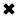# Wattmeters Explained

Wattmeters

The measurement of electrical power requires that voltage and current both be mea­sured simultaneously. Remember that power is the product of the voltage and current. That is, watts (P) equals volts (E) times amperes (I), written as P = El. In fact, watts are sometimes called volt-amperes in a dc circuit.

• Wattmeters are instruments used to measure electrical power.
• The traditional form of wattmeters are called "dynamometer wattmeters"
• Wattmeters are available as digital wattmeters

Wattmeter Construction & Working Principle:

• The dynamometer wattmeter works on the motor principle.
• As shown in the below, the wattmeter has two sets of coils.
• One coil is fixed and is made in two identical parts.  It is made upon heavy gauge copper wire. So it has low resistance. This is named as current coil.
• The other coil which is known as voltage coil,  is wound from fine gauge wire. So it has relatively high resistance. The voltage coil is mounted on a circular manner. It is placed between the two parts of the current coil.You might think that you can just connect a voltmeter in parallel with a circuit, thereby getting a reading of the voltage across it, and also hook up an ammeter in series to get a reading of the current through the circuit, and then multiply volts times am­peres to get watts consumed by the circuit. And in fact, for practically all dc circuits, this is an excellent way to measure power (Fig. 3-10).

Quite often, however, it's simpler than that. In many cases, the voltage from the power supply is constant and predictable. Utility power is a good example. The effec­tive voltage is always very close to 117 V. Although it's ac, and not dc, power can be mea­sured in the same way as with dc: by means of an ammeter connected in series with the circuit, and calibrated so that the multiplication (times 117) has already been done. Then, rather than 1 A, the meter would show a reading of 117 W, because P = El = 117 X 1 = 117 W. If the meter reading were 300 W, the current would be 300/117 = 2.56 A.

An electric iron might consume 1000 W, or a current of 1000/117 = 8.55 A. And a large heating unit might gobble up 2000 W, requiring a current of 2000/117 = 17.1 A. This might blow a fuse or breaker, since these devices are often rated for only 15 A. You've probably had an experience where you hooked up too many appliances to a single circuit, blowing the fuse or breaker. The reason was that the appliances, combined, drew too much current for the house wiring to safely handle, and the fuse or breaker, detecting the excess current, opened the circuit.

Specialized wattmeters are necessary for the measurement of radio-frequency (RF) power, or for peak audio power in a high-fidelity amplifier, or for certain other spe­cialized applications. But almost all of these meters, whatever the associated circuitry, use simple ammeters as their indicating devices.Fig. 3-10 Power can be measured with a voltmeter and an ammeter.

##### Basic Electricity Handbook, Vol. 1• FULLY ILLUSTRATED
• GREAT FOR SCHOOL PROJECTS
• GREAT PRICE: \$5.99
• ...

This 100+ page e-book is a great guide for those who have a basic interest in the field of electricity. This well-illustrated e-book, coupled with some basic knowledge of electricity, will give you a broad theoretical background in this fundamental subject.

CONTENTS
• FUNDAMENTALS OF ELECTRICITY
• HOW DOES ELECTRICITY WORK?
• HOW IS ELECTRICITY GENERATED?
• WHERE DOES ELECTRICITY COME FROM?
• BASIC HOME WIRING
• ELECTRICITY FOR STUDENTS
• ENERGY SAVING TIPS

##### TRAINING EF COURSES

Content Community Connection
Top